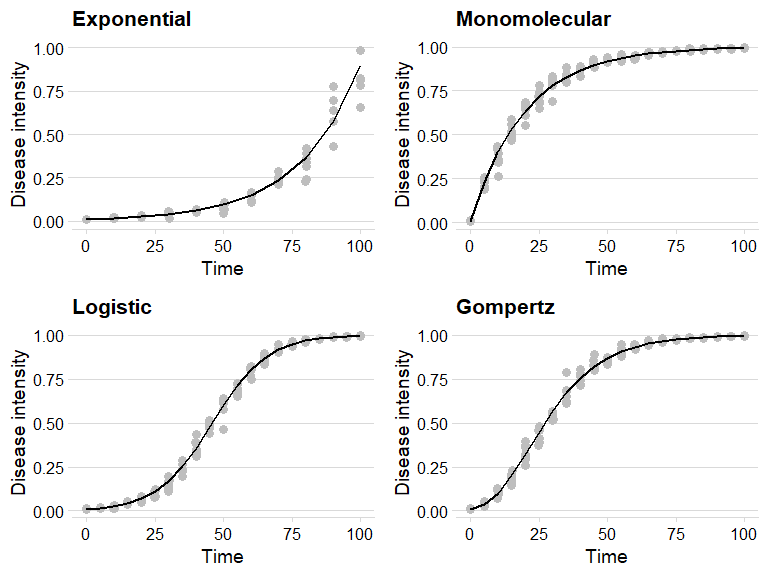# Introduction

In epiffiter, opposite to the fit_ functions (estimate parameters from fitting models to the data), the sim_ family of functions allows to produce the DPC data given a set of parameters for a specific model. Currently, the same four population dynamic models that are fitted to the data can be simulated.

The functions use the ode() function of the devolve package (Soetaert,Petzoldt & Setzer 2010) to solve the differential equation form of the e epidemiological models.

# Hands On

## Packages

First, we need to load the packages we’ll need for this tutorial.

library(epifitter)
library(magrittr)
library(ggplot2)
library(cowplot)

## The basics of the simulation

The sim_ functions, regardless of the model, require the same set of six arguments. By default, at least two arguments are required (the others have default values)

• r: apparent infection rate
• n: number of replicates

When n is greater than one, replicated epidemics (e.g. replicated treatments) are produced and a level of noise (experimental error) should be set in the alpha argument. These two arguments combined set will generate random_y values, which will vary randomly across the defined number of replicates.

The other arguments are:

• N: epidemic duration in time units
• dt: time (fixed) in units between two assessments
• y0: initial inoculum
• alpha: noise parameters for the replicates

### Exponential

Let’s simulate a curve resembling the exponential growth.

exp_model <- sim_exponential(
N = 100,    # total time units
y0 = 0.01,  # initial inoculum
dt = 10,    #  interval between assessments in time units
r = 0.045,  #  apparent infection rate
alpha = 0.2,# level of noise
n = 7       # number of replicates
)
head(exp_model)
##   replicates time          y   random_y
## 1          1    0 0.01000000 0.01194411
## 2          1   10 0.01568425 0.01456530
## 3          1   20 0.02459905 0.02571672
## 4          1   30 0.03858028 0.04925098
## 5          1   40 0.06050749 0.06666500
## 6          1   50 0.09489670 0.09481374

A data.frame object is produced with four columns:

• replicates: the curve with the respective ID number
• time: the assessment time
• y: the simulated proportion of disease intensity
• random_y: randomly simulated proportion disease intensity based on the noise

Use the ggplot2 package to build impressive graphics!

exp_plot = exp_model %>%
ggplot(aes(time, y)) +
geom_jitter(aes(time, random_y), size = 3,color = "gray", width = .1) +
geom_line(size = 1) +
theme_minimal_hgrid() +
ylim(0,1)+
labs(
title = "Exponential",
y = "Disease intensity",
x = "Time"
)
exp_plot
## Warning: Removed 1 rows containing missing values (geom_point).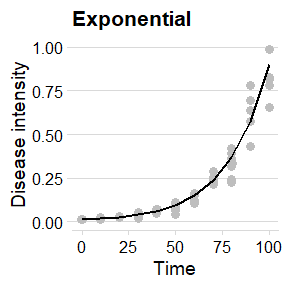### Monomolecular

The logic is exactly the same here.

mono_model <- sim_monomolecular(
N = 100,
y0 = 0.01,
dt = 5,
r = 0.05,
alpha = 0.2,
n = 7
)
head(mono_model)
##   replicates time         y   random_y
## 1          1    0 0.0100000 0.01068644
## 2          1    5 0.2289861 0.23004478
## 3          1   10 0.3995322 0.41563728
## 4          1   15 0.5323535 0.46728941
## 5          1   20 0.6357949 0.61329989
## 6          1   25 0.7163551 0.67702267
mono_plot = mono_model %>%
ggplot(aes(time, y)) +
geom_jitter(aes(time, random_y), size = 3, color = "gray", width = .1) +
geom_line(size = 1) +
theme_minimal_hgrid() +
labs(
title = "Monomolecular",
y = "Disease intensity",
x = "Time"
)
mono_plot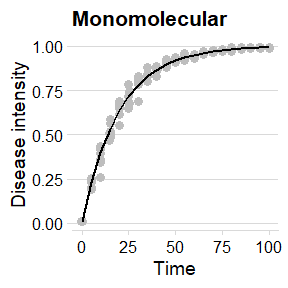### The Logistic model

logist_model <- sim_logistic(
N = 100,
y0 = 0.01,
dt = 5,
r = 0.1,
alpha = 0.2,
n = 7
)
head(logist_model)
##   replicates time          y   random_y
## 1          1    0 0.01000000 0.01213001
## 2          1    5 0.01638216 0.01260491
## 3          1   10 0.02672677 0.01500071
## 4          1   15 0.04331509 0.04050633
## 5          1   20 0.06946352 0.07952141
## 6          1   25 0.10958806 0.07969412
logist_plot = logist_model %>%
ggplot(aes(time, y)) +
geom_jitter(aes(time, random_y), size = 3,color = "gray", width = .1) +
geom_line(size = 1) +
theme_minimal_hgrid() +
labs(
title = "Logistic",
y = "Disease intensity",
x = "Time"
)
logist_plot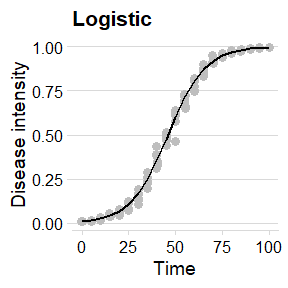### Gompertz

gomp_model <- sim_gompertz(
N = 100,
y0 = 0.01,
dt = 5,
r = 0.07,
alpha = 0.2,
n = 7
)
head(gomp_model)
##   replicates time          y   random_y
## 1          1    0 0.01000000 0.01140562
## 2          1    5 0.03896283 0.03124351
## 3          1   10 0.10158896 0.11865503
## 4          1   15 0.19958740 0.17189356
## 5          1   20 0.32122825 0.30391275
## 6          1   25 0.44922018 0.46034387
gomp_plot = gomp_model %>%
ggplot(aes(time, y)) +
geom_jitter(aes(time, random_y), size = 3,color = "gray", width = .1) +
geom_line(size = 1) +
theme_minimal_hgrid() +
labs(
title = "Gompertz",
y = "Disease intensity",
x = "Time"
)
gomp_plot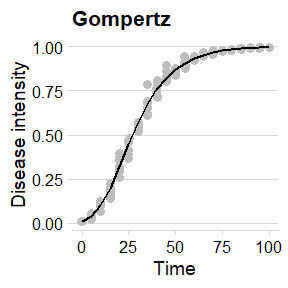## Combo

Use the function plot_grid() from the cowplot package to gather all plots into a grid

plot_grid(exp_plot,
mono_plot,
logist_plot,
gomp_plot)
## Warning: Removed 1 rows containing missing values (geom_point).